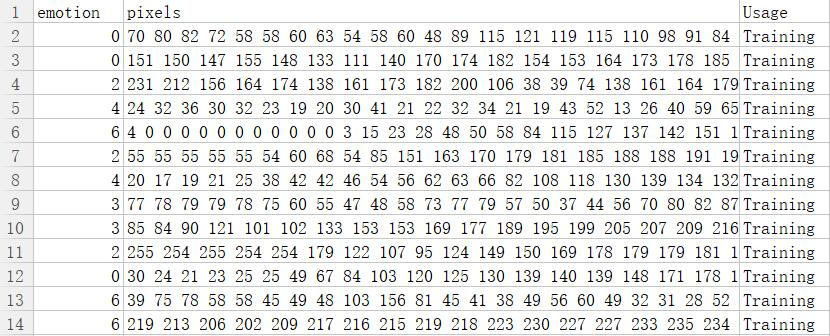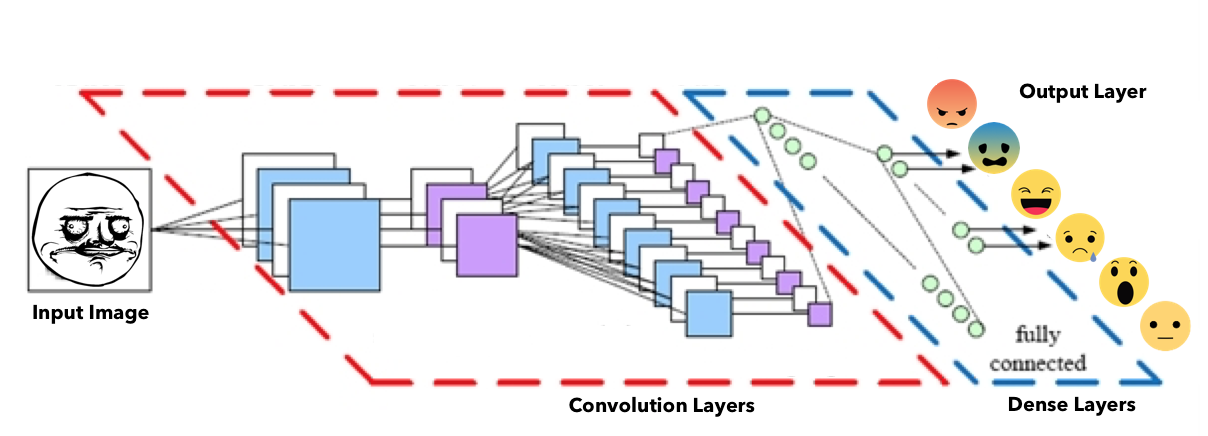• EnergyNews ...程序能将各种新闻进行情绪分类并展示标题和图片，可以根据自己的兴趣选择查看相应情绪类别的新闻。点击图片能够链接到对应的新网页。山下滑动改变情绪类别，左右滑动查看相同类别的新闻。 *Java
• 但是表情识别很难，因为人脸的微表情很多，本节介绍一种比较粗线条的表情分类与识别的办法。 Keras系列：keras系列︱Sequential与Model模型、keras基本结构功能（一） keras系列︱Application中五款已训练模型、VGG...

人脸识别热门，表情识别更加。但是表情识别很难，因为人脸的微表情很多，本节介绍一种比较粗线条的表情分类与识别的办法。

Keras系列：

1、keras系列︱Sequential与Model模型、keras基本结构功能（一）
2、keras系列︱Application中五款已训练模型、VGG16框架（Sequential式、Model式）解读（二）
3、keras系列︱图像多分类训练与利用bottleneck features进行微调（三）
4、keras系列︱人脸表情分类与识别：opencv人脸检测+Keras情绪分类（四）
5、keras系列︱迁移学习：利用InceptionV3进行fine-tuning及预测、完整案例（五）

本次讲述的表情分类是识别的分析流程分为：

1、加载pre-model网络与权重；
2、利用opencv的函数进行简单的人脸检测；
3、抠出人脸的图并灰化；
4、表情分类器检测
.

一、表情数据集

主要来源于kaggle比赛，下载地址。
有七种表情类别： (0=Angry, 1=Disgust, 2=Fear, 3=Happy, 4=Sad, 5=Surprise, 6=Neutral).
数据是48x48 灰度图，格式比较奇葩。
第一列是情绪分类，第二列是图像的numpy，第三列是train or test。.

二、opencv的人脸识别

参考《opencv+Recorder︱OpenCV 中使用 Haar 分类器进行面部检测》
理论略过，直接来看重点：
（1）加载人脸检测器，haarcascade_frontalface_default.xml；
（2）图片加载并灰化，cvtColor，可参考： opencv︱图像的色彩空間cvtColor（HSV、HSL、HSB 、BGR）
（2）人脸探测，detectMultiScale.

# （1）加载人脸检测器
cascPath = '/.../haarcascade_frontalface_default.xml'
faceCascade = cv2.CascadeClassifier(cascPath)

# （2）图片加载并灰化
jpg_file = '/home/ubuntu/keras/image/8c80abb4gw1f3b5hxd3aaj20jg0cx411.jpg'
img_gray = cv2.imread(jpg_file)
img_gray = cv2.cvtColor(img_gray, cv2.COLOR_BGR2GRAY)

# 人脸探测
faces = faceCascade.detectMultiScale(
img_gray,
scaleFactor=1.1,
minNeighbors=1,# minNeighbors=5比较难检测
minSize=(30, 30),
flags=cv2.cv.CV_HAAR_SCALE_IMAGE
)

其中minNeighbors设置小一些，容易检测出来。这个检测器还是有点粗糙。
.

三、表情分类与识别

本节源自github的mememoji。
网络结构：opencv中的人脸检测的pre-model文件（haarcascade_frontalface_default.xml）和表情识别pre-model文件（model.h5）都在作者的github下载。

是利用Keras实现的。直接来看完整的代码：

import cv2
import sys
import json
import time
import numpy as np
from keras.models import model_from_json

emotion_labels = ['angry', 'fear', 'happy', 'sad', 'surprise', 'neutral']

# load json and create model arch
json_file = open('/.../model.json','r')
loaded_model_json = json_file.read()
json_file.close()
model = model_from_json(loaded_model_json)

# load weights into new model
model.load_weights('/.../model.h5')

def predict_emotion(face_image_gray): # a single cropped face
resized_img = cv2.resize(face_image_gray, (48,48), interpolation = cv2.INTER_AREA)
# cv2.imwrite(str(index)+'.png', resized_img)
image = resized_img.reshape(1, 1, 48, 48)
list_of_list = model.predict(image, batch_size=1, verbose=1)
angry, fear, happy, sad, surprise, neutral = [prob for lst in list_of_list for prob in lst]
return [angry, fear, happy, sad, surprise, neutral]

# -------------------直接预测-----------------------
img_gray = cv2.imread('/.../real-time_emotion_analyzer-master/meme_faces/angry-angry.png')
img_gray = cv2.cvtColor(img_gray, cv2.COLOR_BGR2GRAY)
angry, fear, happy, sad, surprise, neutral = predict_emotion(img_gray)

# -------------------人脸预测-----------------------
# 加载检测器
cascPath = '/.../real-time_emotion_analyzer-master/haarcascade_frontalface_default.xml'
faceCascade = cv2.CascadeClassifier(cascPath)

# 图像灰化
jpg_file = '/.../001.jpg'
img_gray = cv2.imread(jpg_file)
img_gray = cv2.cvtColor(img_gray, cv2.COLOR_BGR2GRAY)

# 人脸检测
faces = faceCascade.detectMultiScale(
img_gray,
scaleFactor=1.1,
minNeighbors=1,# minNeighbors=5比较难检测
minSize=(30, 30),
flags=cv2.cv.CV_HAAR_SCALE_IMAGE
)

# 表情画框
for (x, y, w, h) in faces:
face_image_gray = img_gray[y:y+h, x:x+w]
cv2.rectangle(frame, (x, y), (x+w, y+h), (0, 255, 0), 2)
angry, fear, happy, sad, surprise, neutral = predict_emotion(face_image_gray)
展开全文脸部识别 opencv 表情识别 keras
• 本次讲述的表情分类是识别的分析流程分为...3、抠出人脸的并灰化； 4、表情分类器检测 . 一、表情数据集 主要来源于kaggle比赛，下载地址。 有七种表情类别： (0=Angry, 1=Disgust, 2=Fear, 3=Happy, 4=Sad, 5...


本次讲述的表情分类是识别的分析流程分为：
1、加载pre-model网络与权重；
2、利用opencv的函数进行简单的人脸检测；
3、抠出人脸的图并灰化；
4、表情分类器检测
.

一、表情数据集
主要来源于kaggle比赛，下载地址。
有七种表情类别： (0=Angry, 1=Disgust, 2=Fear, 3=Happy, 4=Sad, 5=Surprise, 6=Neutral).
数据是48x48 灰度图，格式比较奇葩。
第一列是情绪分类，第二列是图像的numpy，第三列是train or test。.
二、opencv的人脸识别
参考《opencv+Recorder︱OpenCV 中使用 Haar 分类器进行面部检测》
理论略过，直接来看重点：
（1）加载人脸检测器，haarcascade_frontalface_default.xml；
（2）图片加载并灰化，cvtColor，可参考： opencv︱图像的色彩空間cvtColor（HSV、HSL、HSB 、BGR）
（2）人脸探测，detectMultiScale.
# （1）加载人脸检测器
cascPath = '/.../haarcascade_frontalface_default.xml'
faceCascade = cv2.CascadeClassifier(cascPath)

# （2）图片加载并灰化
jpg_file = '/home/ubuntu/keras/image/8c80abb4gw1f3b5hxd3aaj20jg0cx411.jpg'
img_gray = cv2.imread(jpg_file)
img_gray = cv2.cvtColor(img_gray, cv2.COLOR_BGR2GRAY)

# 人脸探测
faces = faceCascade.detectMultiScale(
img_gray,
scaleFactor=1.1,
minNeighbors=1,# minNeighbors=5比较难检测
minSize=(30, 30),
flags=cv2.cv.CV_HAAR_SCALE_IMAGE
)

1
2
3
4
5
6
7
8
9
10
11
12
13
14
15
16
17
其中minNeighbors设置小一些，容易检测出来。这个检测器还是有点粗糙。
.

三、表情分类与识别
本节源自github的mememoji。
网络结构：opencv中的人脸检测的pre-model文件（haarcascade_frontalface_default.xml）和表情识别pre-model文件（model.h5）都在作者的github下载。

是利用Keras实现的。直接来看完整的代码：
import cv2
import sys
import json
import time
import numpy as np
from keras.models import model_from_json

emotion_labels = ['angry', 'fear', 'happy', 'sad', 'surprise', 'neutral']

# load json and create model arch
json_file = open('/.../model.json','r')
loaded_model_json = json_file.read()
json_file.close()
model = model_from_json(loaded_model_json)

# load weights into new model
model.load_weights('/.../model.h5')

def predict_emotion(face_image_gray): # a single cropped face
resized_img = cv2.resize(face_image_gray, (48,48), interpolation = cv2.INTER_AREA)
# cv2.imwrite(str(index)+'.png', resized_img)
image = resized_img.reshape(1, 1, 48, 48)
list_of_list = model.predict(image, batch_size=1, verbose=1)
angry, fear, happy, sad, surprise, neutral = [prob for lst in list_of_list for prob in lst]
return [angry, fear, happy, sad, surprise, neutral]

# -------------------直接预测-----------------------
img_gray = cv2.imread('/.../real-time_emotion_analyzer-master/meme_faces/angry-angry.png')
img_gray = cv2.cvtColor(img_gray, cv2.COLOR_BGR2GRAY)
angry, fear, happy, sad, surprise, neutral = predict_emotion(img_gray)

# -------------------人脸预测-----------------------
# 加载检测器
cascPath = '/.../real-time_emotion_analyzer-master/haarcascade_frontalface_default.xml'
faceCascade = cv2.CascadeClassifier(cascPath)

# 图像灰化
jpg_file = '/.../001.jpg'
img_gray = cv2.imread(jpg_file)
img_gray = cv2.cvtColor(img_gray, cv2.COLOR_BGR2GRAY)

# 人脸检测
faces = faceCascade.detectMultiScale(
img_gray,
scaleFactor=1.1,
minNeighbors=1,# minNeighbors=5比较难检测
minSize=(30, 30),
flags=cv2.cv.CV_HAAR_SCALE_IMAGE
)

# 表情画框
for (x, y, w, h) in faces:
face_image_gray = img_gray[y:y+h, x:x+w]
cv2.rectangle(frame, (x, y), (x+w, y+h), (0, 255, 0), 2)
angry, fear, happy, sad, surprise, neutral = predict_emotion(face_image_gray)

from:https://blog.csdn.net/sinat_26917383/article/details/72885715


展开全文• 向AI转型的程序员都关注了这个号???大数据挖掘DT数据分析 公众号： datadw脸识别热门，表情识别更加。但是表情识别很难，因为人脸的微表情...3、抠出人脸的并灰化；4、表情分类器检测一、表情数据集主要来源于kaggle向AI转型的程序员都关注了这个号???大数据挖掘DT数据分析  公众号： datadw脸识别热门，表情识别更加。但是表情识别很难，因为人脸的微表情很多，本节介绍一种比较粗线条的表情分类与识别的办法。本次讲述的表情分类是识别的分析流程分为：1、加载pre-model网络与权重；2、利用opencv的函数进行简单的人脸检测；3、抠出人脸的图并灰化；4、表情分类器检测一、表情数据集主要来源于kaggle比赛，下载地址。 在公众号 datadw 里 回复 keras   即可获取。有七种表情类别： (0=Angry, 1=Disgust, 2=Fear, 3=Happy, 4=Sad, 5=Surprise, 6=Neutral). 数据是48x48 灰度图，格式比较奇葩。 第一列是情绪分类，第二列是图像的numpy，第三列是train or test。二、opencv的人脸识别参考《opencv+Recorder︱OpenCV 中使用 Haar 分类器进行面部检测》 http://blog.csdn.net/sinat_26917383/article/details/69831495理论略过，直接来看重点： （1）加载人脸检测器，haarcascade_frontalface_default.xml； （2）图片加载并灰化，cvtColor，可参考： opencv︱图像的色彩空間cvtColor（HSV、HSL、HSB 、BGR） http://blog.csdn.net/sinat_26917383/article/details/70860910（2）人脸探测，detectMultiScale.# （1）加载人脸检测器cascPath = '/.../haarcascade_frontalface_default.xml'faceCascade = cv2.CascadeClassifier(cascPath)# （2）图片加载并灰化jpg_file = '/home/ubuntu/keras/image/8c80abb4gw1f3b5hxd3aaj20jg0cx411.jpg'img_gray = cv2.imread(jpg_file)img_gray = cv2.cvtColor(img_gray, cv2.COLOR_BGR2GRAY)# 人脸探测faces = faceCascade.detectMultiScale(        img_gray,        scaleFactor=1.1,        minNeighbors=1,# minNeighbors=5比较难检测        minSize=(30, 30),        flags=cv2.cv.CV_HAAR_SCALE_IMAGE    )其中minNeighbors设置小一些，容易检测出来。这个检测器还是有点粗糙。三、表情分类与识别本节源自github的mememoji。 https://github.com/JostineHo/mememoji网络结构：opencv中的人脸检测的pre-model文件（haarcascade_frontalface_default.xml）和表情识别pre-model文件（model.h5）都在作者的github下载。作者的github地址在公众号 datadw 里 回复 keras   即可获取。是利用Keras实现的。直接来看完整的代码：import cv2import sysimport jsonimport timeimport numpy as npfrom keras.models import model_from_jsonemotion_labels = ['angry', 'fear', 'happy', 'sad', 'surprise', 'neutral']# load json and create model archjson_file = open('/.../model.json','r')loaded_model_json = json_file.read()json_file.close()model = model_from_json(loaded_model_json)# load weights into new modelmodel.load_weights('/.../model.h5')def predict_emotion(face_image_gray): # a single cropped face    resized_img = cv2.resize(face_image_gray, (48,48), interpolation = cv2.INTER_AREA)    # cv2.imwrite(str(index)+'.png', resized_img)    image = resized_img.reshape(1, 1, 48, 48)    list_of_list = model.predict(image, batch_size=1, verbose=1)    angry, fear, happy, sad, surprise, neutral = [prob for lst in list_of_list for prob in lst]    return [angry, fear, happy, sad, surprise, neutral]# -------------------直接预测-----------------------img_gray = cv2.imread('/.../real-time_emotion_analyzer-master/meme_faces/angry-angry.png')img_gray = cv2.cvtColor(img_gray, cv2.COLOR_BGR2GRAY)angry, fear, happy, sad, surprise, neutral = predict_emotion(img_gray)# -------------------人脸预测-----------------------# 加载检测器cascPath = '/.../real-time_emotion_analyzer-master/haarcascade_frontalface_default.xml'faceCascade = cv2.CascadeClassifier(cascPath)# 图像灰化jpg_file = '/.../001.jpg'img_gray = cv2.imread(jpg_file)img_gray = cv2.cvtColor(img_gray, cv2.COLOR_BGR2GRAY)# 人脸检测faces = faceCascade.detectMultiScale(        img_gray,        scaleFactor=1.1,        minNeighbors=1,# minNeighbors=5比较难检测        minSize=(30, 30),        flags=cv2.cv.CV_HAAR_SCALE_IMAGE    )# 表情画框for (x, y, w, h) in faces:    face_image_gray = img_gray[y:y+h, x:x+w]    cv2.rectangle(frame, (x, y), (x+w, y+h), (0, 255, 0), 2)    angry, fear, happy, sad, surprise, neutral = predict_emotion(face_image_gray)via : http://blog.csdn.net/sinat_26917383/article/details/72885715人工智能大数据与深度学习搜索添加微信公众号：weic2c长按图片，识别二维码，点关注大数据挖掘DT数据分析搜索添加微信公众号：datadw教你机器学习，教你数据挖掘长按图片，识别二维码，点关注


展开全文• 如果有，它将根据六种可能的情绪进行分类：悲伤，愤怒，惊奇，幸福，厌恶，恐惧。 Microsoft Cognitive Services已被利用来从训练和测试集图像中提取标签，并使用一揽子单词模型（我们采用术语频率）创建矢量空间，...PHP
• 基于正则化神经网络的脑电情绪识别 一、 引言（Introduction） 论文动机 ​ 现有的基于脑电图的情绪识别方法大多不能很好地解决以下三个方面的问题: 1)脑电图信号的拓扑结构没有被有效地利用来学习更多的有鉴别性...
基于正则化图神经网络的脑电情绪识别一、 引言（Introduction）
论文动机
​       现有的基于脑电图的情绪识别方法大多不能很好地解决以下三个方面的问题: 1)脑电图信号的拓扑结构没有被有效地利用来学习更多的有鉴别性的脑电图特征然而，大多数方法需要在头皮上对脑电图通道进行2D表示，这可能会在flatten过程中造成信息丢失，因为通道实际上是在3D空间中排列的; 2)不同受试者的脑电图信号差异较大，不利于训练分类器的泛化; 3)参与者在观看引发情绪的刺激时，可能并不总是产生与刺激一致的预期情绪。目前研究中，在基于脑电图的情绪识别方面，还没有人试图解决噪音标签的问题。作者提出了一个正则化的图神经网络(RGNN)来解决上述三个问题。
论文工作
​        脑电信号中的每个通道看作是图形中的一个节点。RGNN模型扩展了简单图卷积网络(SGC)，利用了脑电图信号的拓扑结构，即根据脑网络组织的经济性，作者提出了一个符合生物原理的稀疏邻接矩阵来捕捉局部和全局的通道间关系。局部通道间关系连接附近的神经元群，可以显示解剖学上的连通性。整体的通道间关系连接左右脑半球之间的神经元群，可以揭示与情绪相关的功能连接。此外，作者提出一个节点域对抗训练(NodeDAT)来正则化图模型，以更好地解决跨被试分类场景，并且还提出了一种基于情绪感知的分布学习(EmotionDL)方法来解决数据集中的噪声标签问题。
二、RGNN知识基础
###简单图卷积网络(Simple Graph Convolution Network ,SGC)
​       给定图$\mathcal{G}=(\mathcal{V}, \mathcal{E})$，$\mathcal{V}$表示节点集，$\mathcal{E}$表示边集。$\mathbf{X} \in \mathbb{R}^{n \times d}$表示特征矩阵，$n$表示节点的数目，$d$ 输入特征的维度。 $\mathcal{E}$可以用加权邻接矩阵 $\mathbf{A} \in \mathbb{R}^{n \times n}$表示。 一般情况下，GNNs对输入 $\mathbf{X}$学习一个特征变换函数，生成输出 $\mathbf{Z} \in \mathbb{R}^{n \times d}$,$d$表示输出的维度。特征转换可以写成： $\mathbf{H}^{l+1}=f\left(\mathbf{H}^{l}, \mathbf{A}\right)$，这里 $l=0,1, \ldots, L-1, L$，$\mathbf{H}^{0}=\mathbf{X}, \mathbf{H}^{L}=\mathbf{Z}$。
​       GCN(graph convolution network)的方法如公式所示： $f\left(\mathbf{H}^{l+1}\right)=\sigma\left(\mathbf{D}^{-\frac{1}{2}} \mathbf{A} \mathbf{D}^{\frac{1}{2}} \mathbf{H}^{l} \mathbf{W}^{l}\right)$，其中  $\mathbf{D}$表示 $\mathbf{A}$ 的对角度矩阵，规范化邻接矩阵 $\mathbf{D}^{-\frac{1}{2}} \mathbf{A} \mathbf{D}^{\frac{1}{2}}$可以防止$\mathbf{H}$变得过大。SGC方法消除非线性函数  $\sigma\left(\right)$和将所有层之间的所有线性变换  $\mathbf{W}^{l}$重新参数化为一个线性变换 $\mathbf{W}$，如下所示： $\mathbf{Z}=\mathbf{H}^{L}=\mathbf{S H}^{L-1} \mathbf{W}^{L-1}=\ldots=\mathbf{S}^{L} \mathbf{X} \mathbf{W}$，这里$\mathbf{S}=\mathbf{D}^{-\frac{1}{2}} \mathbf{A D}^{\frac{1}{2}},$  $\mathbf{W}=\mathbf{W}^{L-1} \mathbf{W}^{L-2} \ldots \mathbf{W}^{0}$。本质上SGC计算拓扑感知的线性变换$\hat{\mathbf{X}}=\mathbf{S}^{L} \mathbf{X}$，最后一个线性变换 $\mathbf{Z}=\hat{\mathbf{X}} \mathbf{W}$。
**谱图卷积（**Spectral Graph Convolution）
​       KaTeX parse error: Undefined control sequence: \cal at position 1: \̲c̲a̲l̲ ̲{G}表示为无向连接图，其拉普拉斯矩阵定义为
$L = D - A$
​       $L$ 是laplacian矩阵，$D$是顶点的度矩阵(对角矩阵)，对角线上的元素 $D_{i i}=\sum_{j} A_{i j}$依次为各个顶点的度， $W$是图的邻接矩阵。归一化的定义是 :
$L = {I_n} - {D^{ - 1/2}}A{D^{ - 1/2}} = U\Lambda {U^T}$
其中，拉普拉斯矩阵 $L$被傅里叶基 $U = \left[ {{u_0}, \ldots ,{u_{n - 1}}} \right] \in {^{n \times n}}$对角化，这里， $\Lambda = {\mathop{\rm diag}\nolimits} \left( {\left[ {{\lambda _0}, \ldots ,{\lambda _{n - 1}}} \right]} \right) \in {^{n \times n}}$，${I_n}$是单位矩阵。
​         给定空间信号$x \in \mathbb{R}^{n \times d}$它的图傅里叶变换定义为 $\hat{x}=U^{T} x$，逆变换为 $x=U$ 。在图${G}$上，$x$和 $y$两个信号的卷积表示为：
$x{*_{G}}y = U\left( {\left( {{U^T}x} \right) \odot \left( {{U^T}y} \right)} \right)$
其中，$\odot$表示hadamard product。信号 $x$被${g_\theta }()$滤波输出 $y$可以表示为：
$y = {g_\theta }(L)x = {g_\theta }\left( {U\Lambda {U^T}} \right)x = U{g_\theta }(\Lambda ){U^T}x$
其中， ${g_\theta }(\Lambda )$可以表示为：
$g_{\theta}(\Lambda)=\left[\begin{array}{ccc} g\left(\lambda_{0}\right) & \cdots & 0 \\ \vdots & \ddots & \vdots \\ 0 & \cdots & g\left(\lambda_{N-1}\right) \end{array}\right]$
​       采用$K$阶切比雪夫多项式（Chebyshev polynomials）来代替卷积核的图傅里叶变换 ${g_\theta }(\Lambda )$ 以简化计算复杂度。基于$K$阶切比雪夫多项式， 可以${g_\theta }(\Lambda )$ 近似为：
${g_{{\theta ^\prime }}}(\Lambda ) \approx \sum\limits_{k = 0}^K {\theta _k^\prime {\kern 1pt} } {T_k}(\tilde \Lambda )$
其中，${\theta _k^\prime }$为切比雪夫多项式系数，$\tilde \Lambda = 2\Lambda /{\lambda _{max}} - {I_N}$是标准化的  $\Lambda$标准化后，其对角线元素取值在[-1,1]，$\lambda _{max}$是$\tilde \Lambda$中最大的元素，${I_N}$是N阶单位阵。 ${T_k}(x)$可按以下递推公式计算得到$\left\{\begin{array}{l}T_{0}(x)=1, T_{1}(x)=x \\T_{k}(x)=2 x T_{k-1}(x)-T_{k-2}(x), \quad k \geq 2\end{array}\right.$
​        则对信号 的图滤波操作可以写为： $g_{\theta^{\prime}}(\Lambda) \approx \sum_{k=0}^{K} \theta_{k}^{\prime} T_{k}(\tilde{\Lambda})$一、
三、**论文方法（**Methods）
1.      邻接矩阵 (Adjacency Matrix)图1  62 EEG通道图(灰色对称通道通过红色虚线全局连接)
​      作者在邻接矩阵中初始化局部通道间关系，如下所示： $\mathbf{A}_{i j}=\exp \left(-\frac{d_{i j}}{2 \delta^{2}}\right)$，其中 $d_{i j,} i, j=1,2, \dots, n$表示通道${i ,j}$间的物理距离， $\delta$代表一个稀疏超参数衰变率可以控制通道之间的联系。
​       图1描述了SEED和SEED-IV的全局连接关系，为了利用差分不对称信息，我们将$\mathbf{A}$初始化全局通道间关系到[-1,0]如下: $\mathbf{A}_{i j}=\mathbf{A}_{i j}-1$,邻接矩阵$\mathbf{A}$旨在表示结合局部解剖连接和与情绪相关的整体功能连接的大脑网络。
2.      RGNN图2 RGNN整体框架
图2表示整体RGNN框架，结合NodeDAT和EmotionDL，总体损失函数计算如下： $\Phi^{\prime \prime}=\Phi^{\prime}+\Phi_{D}$，$\Phi^{\prime}, \Phi_{D}$分别为节点域对抗训练与情绪感知分布学习的损失函数。其伪代码如Algorithm 1所示。节点域对抗训练(NodeDAT)
​        $\mathbf{X}^{S} \in \mathbb{R}^{N \times n \times d}$表示给定源域数据，$\mathbf{X}^{T} \in \mathbb{R}^{N \times n \times d}$表示未标记的目标域数据。领域分类器的目标是最小化以下两个二进制交叉熵损失的总和: $\Phi_{D}=-\sum_{i=1}^{N} \sum_{j=1}^{n}\left(\log \left(p_{D}\left(0 | \mathbf{X}_{i}^{S}\right)_{j}+\log \left(p_{D}\left(1 | \mathbf{X}_{i}^{T}\right)_{j}\right)\right)\right.$
​       域分类器的目标是将源数据分类为0，将目标数据分类为1。 点的域概率可以表示为：$\begin{array}{l}\left.p_{D}\left(0 | \mathbf{X}_{i}^{S}\right)\right)_{j}=\operatorname{softmax}_{0}\left(\sigma\left(\mathbf{Z}_{i j}^{S}\right) \mathbf{W}^{D}\right) \\ \left.p_{D}\left(1 | \mathbf{X}_{i}^{T}\right)\right)_{j}=\operatorname{softmax}_{1}\left(\sigma\left(\mathbf{Z}_{i j}^{T}\right) \mathbf{W}^{D}\right)\end{array}$
域分类器利用一个梯度反转层(GRL)来在反向传播期间反转域分类器的梯度。
情绪感知分布学习(EmotionDL)
​         作者转换每个训练样本标签$\mathbf{Y}_{i} \in\{0,1, \ldots, C-1\}$转化为所有类的先验概率分布$\hat{\mathbf{Y}}_{i} \in \mathbb{R}^{\alpha}$。在SEED数据集中，有积极、中性和消极三类情绪，并有相应的类指标分别是0 1 2，将$\mathbf{Y}$转换如下:$\hat{\mathbf{Y}}_{i}=\left\{\begin{array}{ll}\left(1-\frac{2 \epsilon}{3}, \frac{2 \epsilon}{3}, 0\right), & \mathbf{Y}_{i}=0 \\ \left(\frac{\epsilon}{3}, 1-\frac{2 \epsilon}{3}, \frac{\epsilon}{3}\right), & \mathbf{Y}_{i}=1 \\ \left(0, \frac{2 \epsilon}{3}, 1-\frac{2 \epsilon}{3}\right), & \mathbf{Y}_{i}=2\end{array}\right.$
在SEED-IV中，有四个类:中性、悲伤、恐惧和快乐，分别对应的类指标为0、1、2和3，将$\mathbf{Y}$转换如下: $\hat{\mathbf{Y}}_{i}=\left\{\begin{array}{ll}\left(1-\frac{3 \epsilon}{4}, \frac{\epsilon}{4}, \frac{\epsilon}{4}, \frac{\epsilon}{4}\right), & \mathbf{Y}_{i}=0 \\ \left(\frac{\epsilon}{3}, 1-\frac{2 \epsilon}{3}, \frac{\epsilon}{3}, 0\right), & \mathbf{Y}_{i}=1 \\ \left(\frac{\epsilon}{4}, \frac{\epsilon}{4}, 1-\frac{3 \epsilon}{4}, \frac{\epsilon}{4}\right), & \mathbf{Y}_{i}=2 \\ \left(\frac{\epsilon}{3}, 0, \frac{\epsilon}{3}, 1-\frac{2 \epsilon}{3}\right), & \mathbf{Y}_{i}=3\end{array}\right.$
其中， $\epsilon \in [0,1]$表示在训练标签中控制噪音水平的超参数。 在得到转换后的类分布$\mathbf{Y}$ 后，我们的模型可以通过最小化以下Kullback-Leibler (KL)散度来进行优化： $\Phi^{\prime}=\sum_{i=1}^{N} \mathrm{KL}\left(p\left(\mathbf{Y} | \mathbf{X}_{i}, \theta\right), \hat{\mathbf{Y}}_{i}\right)+\alpha\|\mathbf{A}\|_{1}$。
四、 结果（Results）
​        为了评估本文方法的性能，作者在SEED 与SEED-IV脑电图数据集上进行了subject-dependent和subject-independent实验。实验结果如表1与表2所示。RGNN模型在两个数据集上都取得了优于所有基线的性能，包括使用所有频带的DE特征时的最优良的BiHDM模型。除了提出的两个正则化器外，主要的性能改进可以归结于两个因素: 1)邻接矩阵包含了左右半球之间的全局通道间不对称关系; 2)我们的模型通过扩展SGC来减少过拟合的问题，这比DGCNN中使用的ChebNet简单得多。图3邻接矩阵对角元素热力图图4 邻接矩阵A中电极之间的前10个边权值
​       图3中前额叶、顶叶和枕叶区域有强烈的活动，表明这些区域可能与大脑的情感处理有很强的关系。图4显示了邻接矩阵A中，边权值最大的前10个通道之间的连接。注意，在学习A之后，所有的全局连接仍然是最强的连接，这再次证明了全局通道间关系对于情感识别是必不可少的。
五、结论（Conclusion）
。图4显示了邻接矩阵A中，边权值最大的前10个通道之间的连接。注意，在学习A之后，所有的全局连接仍然是最强的连接，这再次证明了全局通道间关系对于情感识别是必不可少的。
五、结论（Conclusion）
​       本文提出了一种基于脑电图信号的正则化图形神经网络。我们的模型在生物学上支持捕获本地和全球渠道间的关系。此外，我们提出了两个正则化器，即NodeDAT和EmotionDL，以提高我们的模型对跨被试情绪识别的鲁棒性。模型分析表明，作者提出的生物支持邻接矩阵和两个正则化器对模型性能做出显著的贡献。对神经元活动的研究表明，前额叶、顶叶和枕叶可能是情感识别中信息量最大的区域。


展开全文深度学习 人工智能
• fer2013情绪分类测试的准确性：66％。 有关更多信息，请参阅 情感/性别示例： 引导式后置道具 实时演示： 机器人团队:) 指示 运行实时情绪演示： python3 video_emotion_color_demo.py 运行实时引导的反向传播...Python
• 尽管人类几乎毫不费力或毫不拖延地识别面部表情，可靠的情绪状态表情识别，但必须教会机器理解面部手势。 ＃＃工具 1 Netbeans IDE 2 Luxand FaceSDK 3 Neuroph – Java 神经网络框架 4 OpenCV 2.4.0 5 JavaCVJava
• 情绪分类应用举例 词嵌入的偏见问题 词汇表征（词嵌入） one-hot 表示：比如词典里有10000个单词，man是第1234个，表示为[0,0...0,1,0...0]（第1234位为1，也表示为O1234）。这种表示法最大缺陷是每个词都...NLP 词嵌入 词向量 自然语言处理
• 情绪模型2. 常用数据集3. 问题难点（挑战）3.1 数据标记困难3.2 情感涉及的图片特征多样化（二）主要方法1. 低级特征2. 中级特征3. 高级特征4. 基于学习的特征（三）论文链接 （一）简介 图片可以用于传达某些情感...神经网络 深度学习 机器学习
• 本文使用卷积神经网络（CNN）对CIFAR-10数据库中的场景进行分类，并在KDEF数据库中检测情绪。 所提出的方法将数据转换到小波域以获得更高的精度和与空间域处理相当的效率。 通过将图像数据划分为子带，重要的特征...
• 由于特征选择是一种组合在最优化问题上，采用基于邻域搜索的改进二元粒子群算法（IBPSO），通过fisher或K最近邻（K-Nearest Neighbor，KNN）分类器，寻找有效的特征来改进情绪状态的分类结果。表明该方法是成功的，...
• 使用Dlib提取人脸地标，并训练多类SVM分类器以识别人脸表情（情感）。 动机： 任务是根据面部表情所显示的情感对人的图像进行分类。 为了训练我们的模型，我们想使用Fer2013 datset，其中包含30,000个表达式图像，...
• 该项目使用Apache Spark生态系统[Spark MLlib + Spark Streaming]在世界地图上实时分析和可视化推文的情绪。 在一个非常高的层次上，该项目概括并涵盖了以下每个广泛的主题： 分布式流处理»Apache Spark 机器...
• 为了实现对受试者情绪状态的分类评估，采用支持向量机(SVM)和基于支持向量机的递归特征筛选(SVM-RFE)算法来筛选参数并设计情绪状态的分类器。结果表明在多种情绪种类图片刺激下，受试者出现了显著的功能响应曲线，...
• 实验1考察了情绪图片的美感效应，表明情绪图片确实能够诱发被试不同风格的美感体验，而经过分类的美感风格图片(优美、喜剧、壮美、悲剧)也能引发被试相应的美感体验；实验2考察了不同美感体验类型在情绪各维度上的...
• 该系统将用于将面部表情分类为基本情绪，即快乐，生气，悲伤，中立和惊奇。 目录 基本信息 可以使用许多不同的方法来克服面部表情识别（FER）的问题，但是最适合自动FER的技术是卷积神经网络（CNN）。 因此，提出了...
• 根据面部图像进行情绪的检测分类因此具有一定挑战性。本课题拟基于图像根据面部语言进行人情绪分类算法研究。在情绪的选择上可以考虑先选择对比性较强的几种例如高兴和悲伤等，在分类结果上可以先不必太细化
• 情感识别和分类是情感计算领域的重要研究内容。 当前的研究集中在视觉和... 在获得不同情绪状态下的脑电波数据后，本文使用AdaBoosting算法等三种统计方法对记录的脑电波数据进行情绪分类。 实验结果表明分类效果良好。
• 本研究涉及来自心理生理信号的个体内部和个体间情绪分类，以及性别和年龄影响及其相互作用对情绪识别的影响的亚组分析。 使用精选的特征优化、分类和评估方法进行个别分类。 亚组分析基于个体间分类。 情绪诱发是...
• 评估模型采用中性情绪刺激下的任务数据作为训练数据，积极情绪和负性情绪刺激下的实验数据作为测试数据，分别取得了92.49%、75.90%和79.99%的平均分类正确率。通过实验数据分析，验证了任务负荷和情绪刺激能够有效...
• 通过VLBP和LBP-TOP算子从三维空间中提取图像序列的肢体动作特征，分析愤怒、无聊、厌恶、恐惧、高兴、疑惑和悲伤七种自然情绪的特点，并用参数优化的支持向量机对情绪分类进行识别，识别率最高能够达到77.0%。...支持向量机
• 采用S5PV210芯片处理器，加载linux2.6.30内核，由USB工业相机获取驾驶员人脸图像，利用一种基于稀疏表示的表情特征数值分类方法，获得驾驶员人脸表情特征数值，最后通过映射获得驾驶员情绪状态结果。当出现预设情况...
• 基于弹性模板匹配的人脸表情识别程序。Gabor小波变换提取人脸表情特征以构造表情弹性，基于弹性模板匹配及K-近邻的分类算法实现人脸表情的识别。 vs2010下运行通过。
• 以视频序列的原始图像、视觉显著图形和叠加的光流图像分别作为三个通道的输入，利用CNN网络对空间特征和局部运动特征进行分析，得到的特征直接输入LSTM网络，进行全局运动特征的学习。最后连接Softmax分类器，对三...卷积神经网络 长短期记忆网络
• 本文着重于使用与人体相关的生理信号，如心电（ECG），脑电图（EEG），肌电（EMG），皮肤电React（GSR），呼吸（RSP），皮肤温度（SKT）对人类情绪识别进行调查。 ）等，以及它们的优缺点。 它还描述了情感计算...
• Tick图表 每一个Tick表都包含了一个bid价和一个ask价格。 线图 所有的图表都是以时间为横轴（X轴），以...前面说道，技术分析认为价格包含所有市场上已知的信息，但是不仅如此，还包含市场情绪信息，例如人们的兴...
• 对于移动应用来讲，主要包括:BLE设备控制和数据传输、多信号数据预处理、数据实时显示、基于基本情感模型的二分类情感识别、用户信息和数据管理、Emotion API的结合、集成的智能交互机器人、历史数据展现、UI交互...
• 通过抓取海量数据，让计算机根据算法定义图片可能表达的情绪标签，之后依靠人类群体智慧帮助计算机识别其中最好的标签，最终，计算机逐步学习如果定义照片的情绪。 Log on to Twitter, Facebook or other social ...
• 最近学会利用Python做了几个词云后，又应用NLP中情感分析，结合snownlp库完成了词云分类，做了积极和消极两类词云，效果如下。 之后我对NLP的知识产生了兴趣，继续深挖，发现王树义老师的这个项目很符合我的口味...NLP Python R...